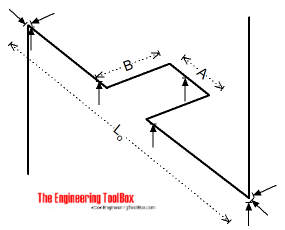Engineering ToolBox - Resources, Tools and Basic Information for Engineering and Design of Technical Applications!

# PVC Pipes - Temperature Expansion Loops

## Calculate temperature expansion and contraction in PVC piping systems.

Temperature expansion and contraction in PVC piping systems can be compensated with

• expansion loops consisting of pipes and 90o elbows
• flexible bends
• bellows and rubber expansion joints
• piston type expansion joints

### Expansion Loops

Expansion loops are made of standard pipes and elbows and can be produced on the site and adapted to the actual situation.The length of the loop can be calculated as

Ll = ((3 E D δl) / (2 S))1/2               (1)

where

Ll  = length of loop (in)

E = modulus of elasticity (psi)

D = outside diameter of pipe (in)

δl = change in pipe length L0 due to temperature change (in)

S = allowable working stress at maximum temperature (psi)

A = Ll / 5           (2)

B = 2 Ll / 5         (3)

Modulus of elasticity for PVC:

• 73oF : 400000 psi
• 100oF : 352000 psi
• 140oF : 280000 psi

Modulus of elasticity for CPVC:

• 73oF : 423000 psi
• 100oF : 385000 psi
• 140oF : 330000 psi
• 200oF : 241000 psi

Maximum working stress for PVC:

• 73oF : 2000 psi
• 100oF : 1240 psi
• 140oF : 440 psi

Maximum working stressfor CPVC:

• 73oF : 2000 psi
• 100oF : 1640 psi
• 140oF : 1000 psi
• 200oF : 400 psi

The temperature expansion of the pipe can be calculated as

δl = α Lo δt            (4)

where

α = expansion coefficient (in/in oF)

Lo = initial length of pipe (ft)

δt = temperature change (oF)

Expansion coefficient for PVC:

• 28 10-6 in/in oF

Expansion coefficient for CPVC:

• 44 10-6 in/in oF

### Example - Expansion Loop

A 2" PVC Schedule 40 straight pipe with outside diameter 2.375 inches and length 300 feet is installed at 70 oF and operated at 140 oF. The expansion coefficient for PVC is 28 10-6 in/in oF.

The expansion of the PVC pipe can from eq. 4 be calculated as

δl = α Lo δt

= (28 10-6 in/in oF) (300 ft) (12 in/ft) ((140 oF) - (70 oF))

= 7.1 inches

The modulus of elasticity is 280000 psi and the maximum working stress is 440 psi at maximum temperature. The length of the loop can from eq. 1 be calculated as

Ll  = ((3 (280000 psi) (2.375 in) (7.1 in)) / (2 (440 psi)))1/2

= 126.5 in

A can be calculated from eq. 2 as

A = (126 in) / 5

= 25.3 in

B can be calculated from eq. 3 as

A = 2 (126 in) / 5

= 50.6 in

## Related Topics

• Temperature Expansion - Thermal expansion of pipes and tubes - stainless steel, carbon steel, copper, plastics and more.

## Engineering ToolBox - SketchUp Extension - Online 3D modeling!

Add standard and customized parametric components - like flange beams, lumbers, piping, stairs and more - to your Sketchup model with the Engineering ToolBox - SketchUp Extension - enabled for use with the amazing, fun and free SketchUp Make and SketchUp Pro .Add the Engineering ToolBox extension to your SketchUp from the SketchUp Pro Sketchup Extension Warehouse!

Translate

## Privacy

We don't collect information from our users. Only emails and answers are saved in our archive. Cookies are only used in the browser to improve user experience.

Some of our calculators and applications let you save application data to your local computer. These applications will - due to browser restrictions - send data between your browser and our server. We don't save this data.

## Citation

• Engineering ToolBox, (2004). PVC Pipes - Temperature Expansion Loops . [online] Available at: https://www.engineeringtoolbox.com/pvc-pipes-expanion-loops-d_805.html [Accessed Day Mo. Year].

Modify access date.

. .

#### Scientific Online Calculator3 30